Question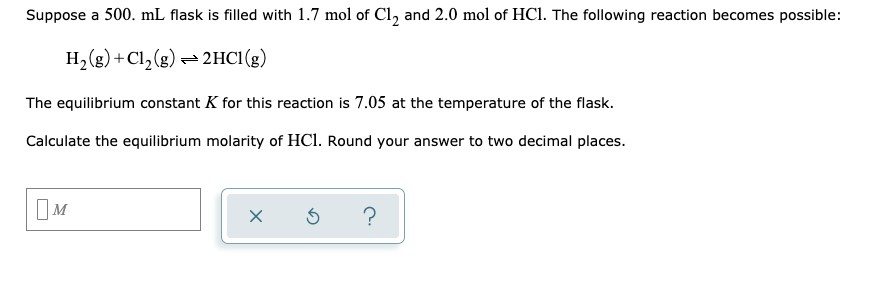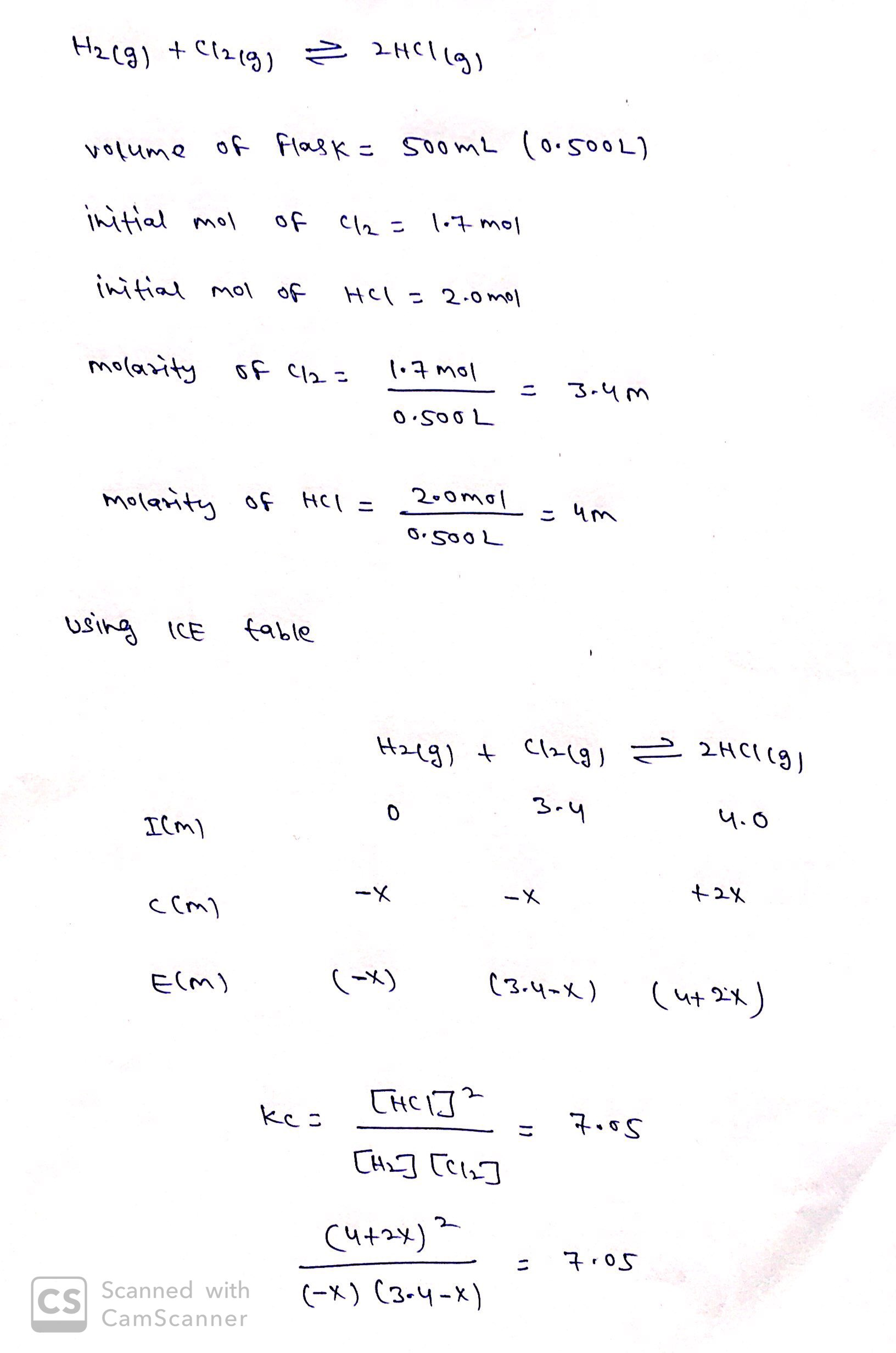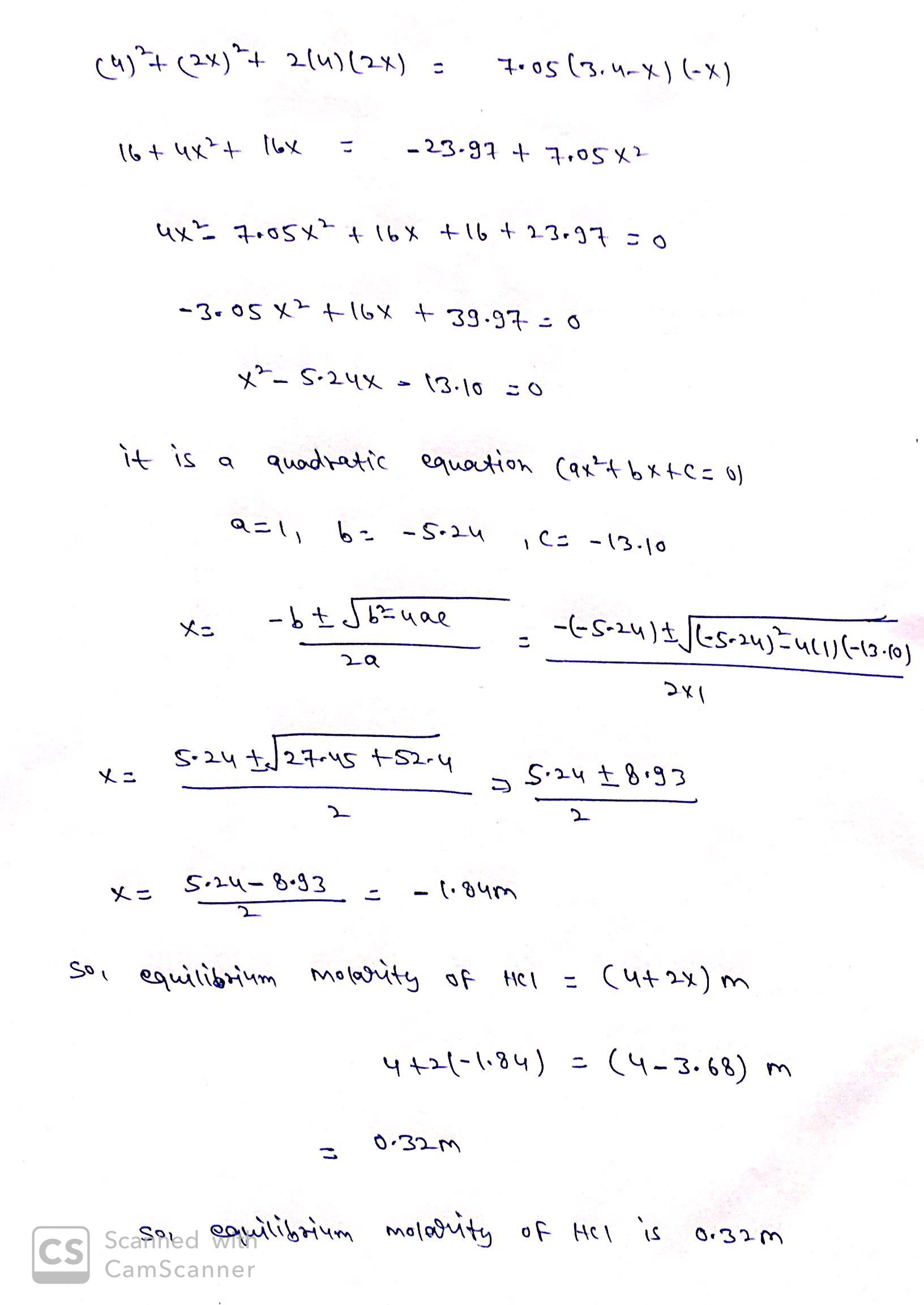#### Earn Coins

Coins can be redeemed for fabulous gifts.

Similar Homework Help Questions
• ### Suppose a 500 ml flask is filled with 1.6 mol of H, and 1.7 mol of...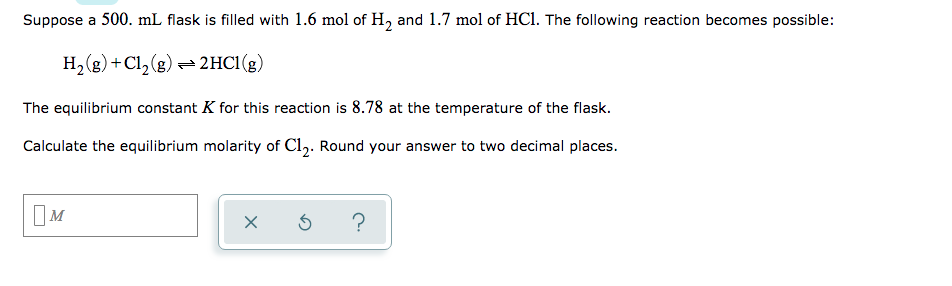Suppose a 500 ml flask is filled with 1.6 mol of H, and 1.7 mol of HCl. The following reaction becomes possible: H2(g) +C12(g) + 2HCI(g) The equilibrium constant K for this reaction is 8.78 at the temperature of the flask. Calculate the equilibrium molarity of Cl,. Round your answer to two decimal places. OM x 6 ?

• ### Suppose a 500. ml flask is filled with 1.2 mol of Cl, and 0.80 mol of...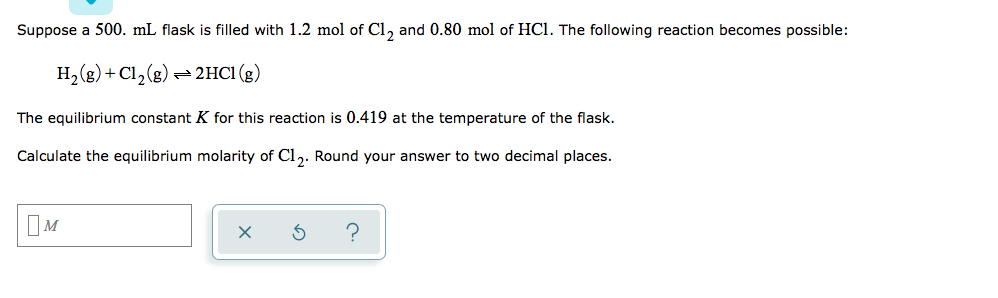Suppose a 500. ml flask is filled with 1.2 mol of Cl, and 0.80 mol of HCl. The following reaction becomes possible: H2(g) + Cl2(g) = 2HCl (g) The equilibrium constant K for this reaction is 0.419 at the temperature of the flask. Calculate the equilibrium molarity of Cl. Round your answer to two decimal places. xs ?

• ### Suppose a 250. ml flask is filled with 1.5 mol of Cl, and 1.3 mol of...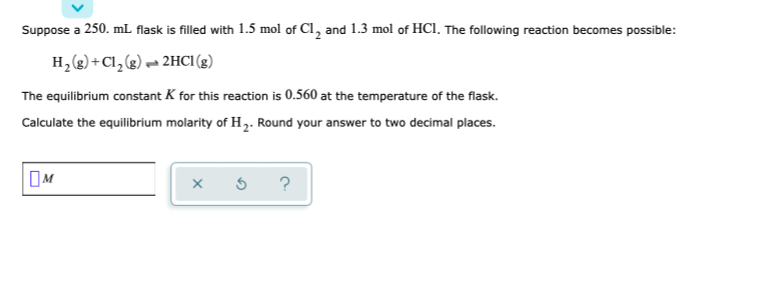Suppose a 250. ml flask is filled with 1.5 mol of Cl, and 1.3 mol of HCl. The following reaction becomes possible: H2(g) + Cl2(g) 2HCl (8) The equilibrium constant K for this reaction is 0.560 at the temperature of the flask. Calculate the equilibrium molarity of H. Round your answer to two decimal places. OM * 5 ?

• ### Suppose a 250. mL flask is filled with 2.0 mol of Cl, 0.20 mol of CHCl,...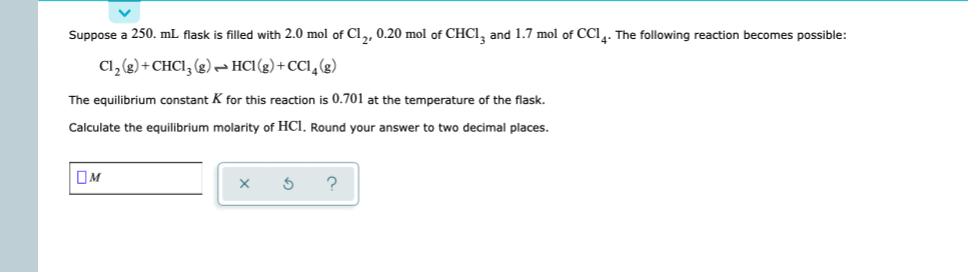Suppose a 250. mL flask is filled with 2.0 mol of Cl, 0.20 mol of CHCl, and 1.7 mol of CCI4. The following reaction becomes possible: Cl2(g) + CHCI, (8) HCI(g) +CCI (8) The equilibrium constant K for this reaction is 0.701 at the temperature of the flask. Calculate the equilibrium molarity of HCl. Round your answer to two decimal places. OM 1 x s ?

• ### Suppose a 500. ml flask is filled with 0.10 mol of H, and 1.0 mol of...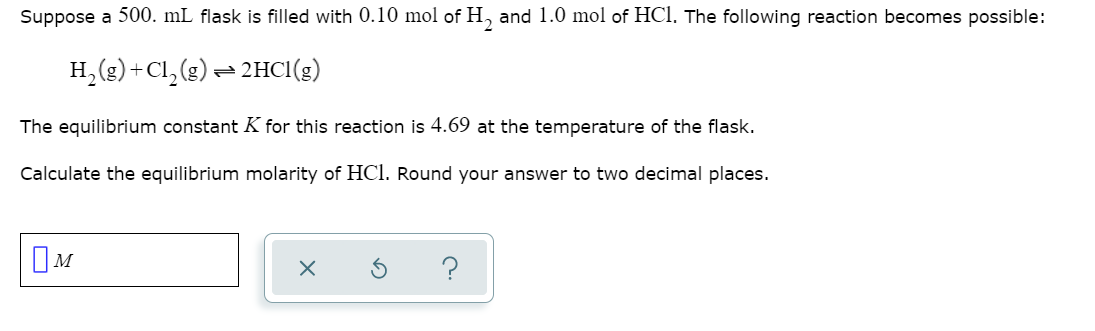Suppose a 500. ml flask is filled with 0.10 mol of H, and 1.0 mol of HCl. The following reaction becomes possible: H2(g) +C1, (g) = 2HCl(g) The equilibrium constant K for this reaction is 4.69 at the temperature of the flask. Calculate the equilibrium molarity of HCl. Round your answer to two decimal places. x 6 ?

• ### Suppose a 500. mL flask is filled with 0.50 mol of H, and 1.7 mol of...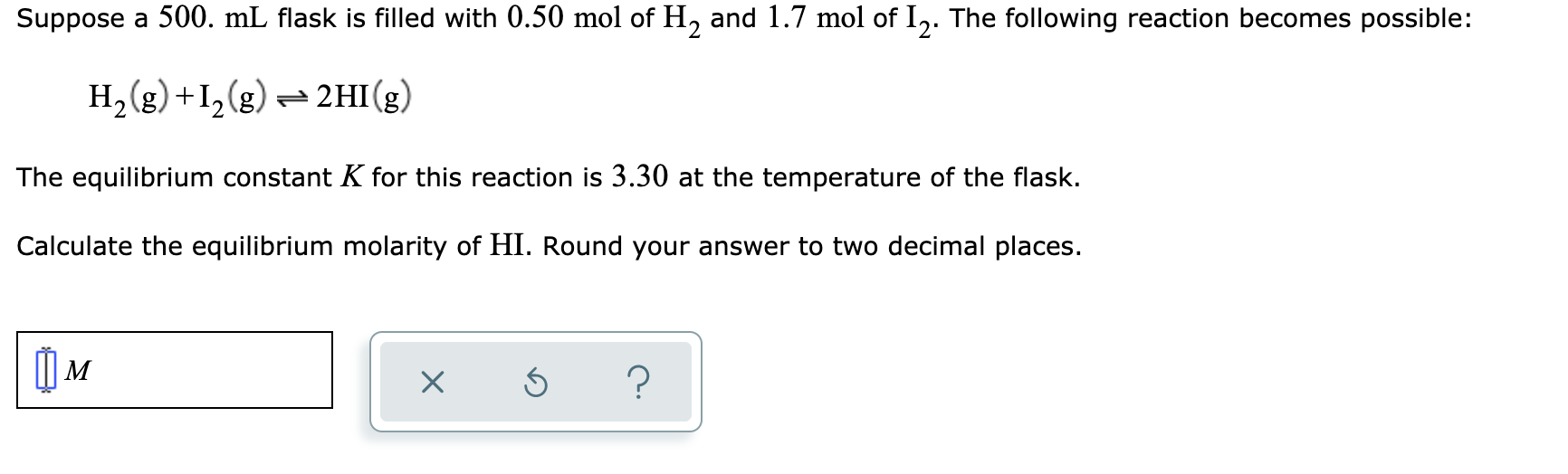Suppose a 500. mL flask is filled with 0.50 mol of H, and 1.7 mol of 12. The following reaction becomes possible: H2(g) +12(g) = 2HI(g) The equilibrium constant K for this reaction is 3.30 at the temperature of the flask. Calculate the equilibrium molarity of HI. Round your answer to two decimal places. xs ?

• ### Suppose a 500. mL flask is filled with 1.3 mol of H2 and 0.10 mol of...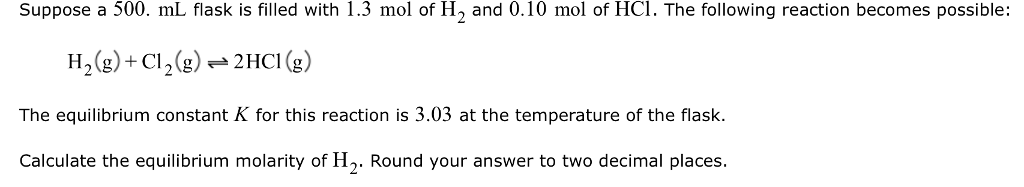Suppose a 500. mL flask is filled with 1.3 mol of H2 and 0.10 mol of HC1. The following reaction becomes possible: H2(g) + Cl2(g)-2HCl (g) The equilibrium constant K for this reaction is 3.03 at the temperature of the flask. Calculate the equilibrium molarity of H2. Round your answer to two decimal places.

• ### Suppose a 250. ml flask is filled with 0.50 mol of H, and 0.40 mol of...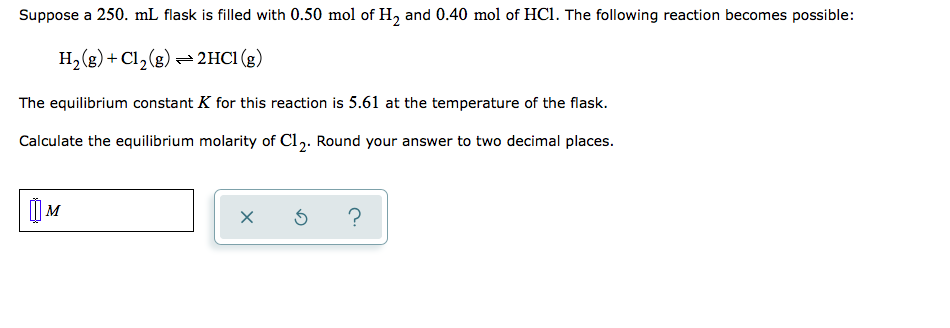Suppose a 250. ml flask is filled with 0.50 mol of H, and 0.40 mol of HCl. The following reaction becomes possible: H2(g) + Cl2(g) = 2HCl (g) The equilibrium constant K for this reaction is 5.61 at the temperature of the flask. Calculate the equilibrium molarity of Cl. Round your answer to two decimal places. TOM xo?

• ### Suppose a 500. mL flask is filled with 0.20 mol of NO2, 1.7 mol of NO...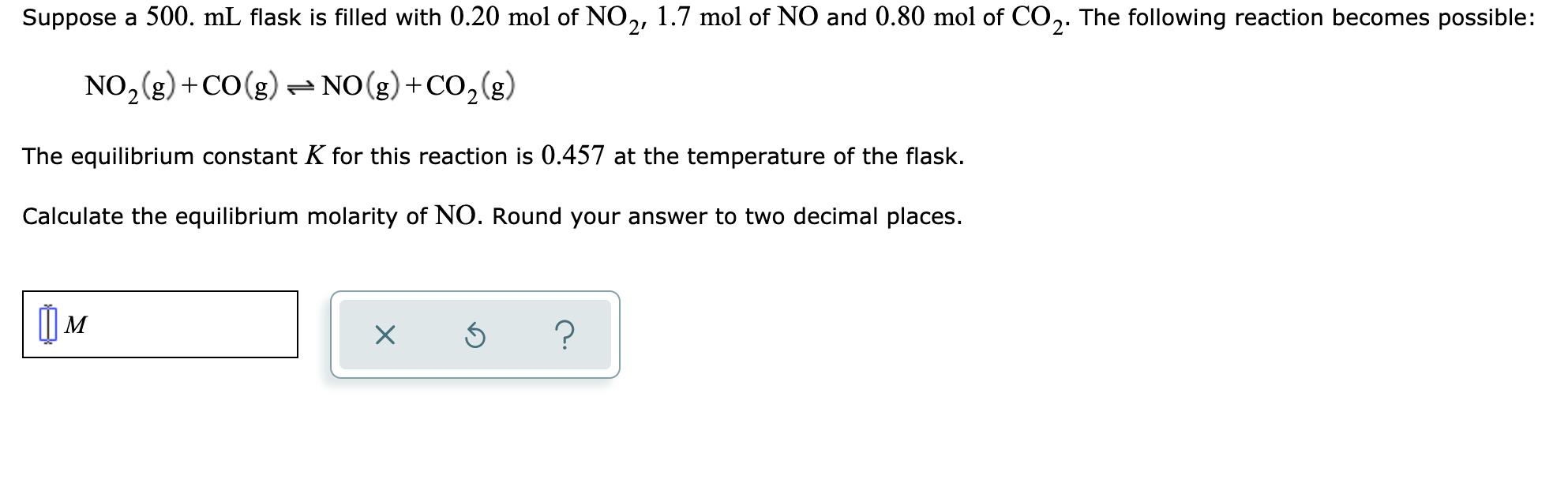Suppose a 500. mL flask is filled with 0.20 mol of NO2, 1.7 mol of NO and 0.80 mol of CO2. The following reaction becomes possible: NO2(g) +CO(g) = NO(g) + CO2(g) The equilibrium constant K for this reaction is 0.457 at the temperature of the flask. Calculate the equilibrium molarity of NO. Round your answer to two decimal places. MM x 6 ?

• ### Suppose a 250. mL flask is filled with 1.2 mol of Cl₂

Suppose a 250. mL flask is filled with 1.2 mol of Cl₂, 1.8 mol of CHCl₃ and 0.90 mol of HCl. The following reaction becomes possible:Cl₂(g)+CHCl₃(g) ⇌ HCl(g)+CCl₄(g)The equilibrium constant K for this reaction is 0.855 at the temperature of the flask.Calculate the equilibrium molarity of HCl. Round your answer to two decimal places.